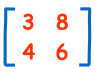Definition of

# DeterminantA special number that can be calculated from a square matrix.

Example: for this matrix the determninant is:
3×6 − 8×4 = 18 − 32 = −14